# Saddle point in game theory

Jump to: navigation, search

A pointof a functiondefined on the Cartesian product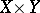of two setsandsuch that(*)

For a functionthe presence of a saddle point is equivalent to the existence of optimal strategies (cf. Strategy (in game theory)) for the players in the two-person zero-sum game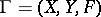.

#### Comments

A point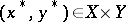satisfying the condition (*) is called a saddle point ofin general. Ifis a differentiable function onand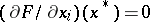,, while the Hessian matrix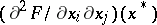is non-singular and neither positive definite nor negative definite, then locally near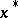,is a saddle point. The corresponding splitting ofnearis determined by the negative and positive eigenspaces of the Hessian at.

Indeed, by the Morse lemma there are coordinates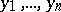nearsuch thathas the form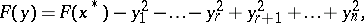whereis the index of the quadratic form determined by the symmetric matrix. (The index of a quadratic form is the dimension of the largest subspace on which it is negative definite; this is also called the negative index of inertia (cf. also Quadratic form and Morse index).)

Let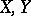be the spaces of strategies of two players in a zero-sum game and letbe (the first component of) the pay-off function (cf. Games, theory of). Then a saddle point is also called an equilibrium point. This notion generalizes to-player non-cooperative games, cf. [a2], Chapt. 2; Games, theory of; Nash theorem (in game theory); Non-cooperative game.